## How to Calculate and Solve for the Radius of Investigation in Well Testing | The Calculator Encyclopedia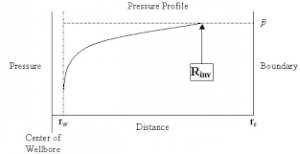The image above represents radius of investigation.

To compute for the radius of investigation, five essential parameters are needed and these parameters are permeability (k), porosity (φ), viscosity (μ), time (t) and total compressibility (CT).

The formula for calculating the radius of investigation:

rinv = 0.0325 √[Kt / φμCT]

Where:

K = Permeability
φ = Porosity
μ = Viscosity
t = Time
CT = Total Compressibility

Let’s solve an example;
Find the radius of investigation when the permeability is 25, porosity is 18, viscosity is 12, time is 22 and total compressibility is 37.

This implies that;

K = Permeability = 25
φ = Porosity =18
μ = Viscosity = 12
t = Time = 22
CT = Total Compressibility = 37

rinv = 0.0325 √[Kt / φμCT]
rinv = 0.0325 √[25 x 22 / 18 x 12 x 37]
rinv = 0.0325 √[25 x 22 / 7992]
rinv = 0.0325 √[550 / 7992]
rinv = 0.0325 √[0.068]
rinv = 0.0325 [0.26]
rinv = 0.0085

Therefore, the radius of investigation is 0.0085 ft.

## How to Calculate and Solve for Infinite Acting Period | The Calculator Encyclopedia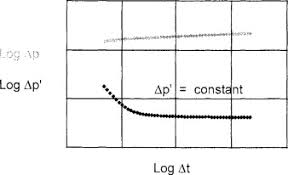The image represents the infinite acting period.

To compute the infinite acting period, six essential parameters are needed and these parameters are permeability (k), porosity (φ), well drainage area (A), viscosity (μ), Dimensionless Time to End of Infinite Acting Period ((tDA)eia) and total compressibility (CT).

The formula for calculating the infinite acting period:

teia = [φ μ CT A / 0.000263K] (tDA)eia

Where;

teia = Infinite Acting Period
K = Permeability
φ = Porosity
A = Well Drainage Area
μ = Viscosity
(tDA)eia = Dimensionless Time to End of Infinite Acting Period
CT = Total Compressibility

Let’s solve an example;
Given that the permeability is 21, porosity is 15, well drainage area is 32, viscosity is 26, dimensionless time to end of infinite acting period is 44 and total compressibility is 34.
Find the infinite acting period?

This implies that;

K = Permeability = 21
φ = Porosity = 15
A = Well Drainage Area = 32
μ = Viscosity = 26
(tDA)eia = Dimensionless Time to End of Infinite Acting Period = 44
CT = Total Compressibility = 34

teia = [φ μ CT A / 0.000263K] (tDA)eia
teia = [15 x 26 x 34 x 32 / 0.000263 x 21] 44
teia = [424320 / 0.005523] 44
teia = [76827810.972] 44
teia = 3380423682.78

Therefore, the infinite acting period is 3380423682.78 s.

## How to Calculate and Solve for Dimensionless Time in Well Testing | The Calculator Encyclopedia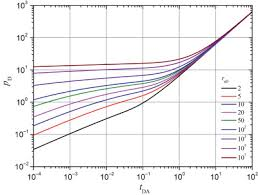The image above represents dimensionless time.

To compute for the dimensionless time, six essential parameters are needed and these parameters are permeability (k), porosity (φ), viscosity (μ), well radius (rw), minimum transient time (t) and total compressibility (CT).

The formula for calculating dimensionless time:

tD = 0.000263kt / φμCTr

Where;

tD = Dimensionless Time
k = Permeability
φ = Porosity
μ = Viscosity
t = Minimum Transient Time
CT = Total Compressibility

Let’s solve an example
Find the dimensionless time when the permeability is 13, porosity is 11.5, viscosity is 10.5, well radius is 24, minimum transient time is 17 with a total compressibility of 28.

This implies that;

k = Permeability = 13
φ = Porosity = 11.5
μ = Viscosity = 10.5
rw = Well Radius = 24
t = Minimum Transient Time = 17
CT = Total Compressibility = 28

tD = 0.000263kt / φμCTr
tD = 0.000263 x 13 x 1711.5 x 10.5 x 28 x 24²
tD = 0.000263 x 13 x 1711.5 x 10.5 x 28 x 576
tD = 0.000263 x 13 x 171947456
tD = 0.0581231947456
tD = 2.9845e-8

Therefore, the dimensionless time is 2.98

Nickzom Calculator – The Calculator Encyclopedia is capable of calculating the dimensionless time.

To get the answer and workings of the dimensionless time using the Nickzom Calculator – The Calculator Encyclopedia. First, you need to obtain the app.

You can get this app via any of these means:

To get access to the professional version via web, you need to register and subscribe for NGN 1,500 per annum to have utter access to all functionalities.
You can also try the demo version via https://www.nickzom.org/calculator

Apple (Paid) – https://itunes.apple.com/us/app/nickzom-calculator/id1331162702?mt=8
Once, you have obtained the calculator encyclopedia app, proceed to the Calculator Map, then click on Petroleum under EngineeringNow, Click on Well Testing under Petroleum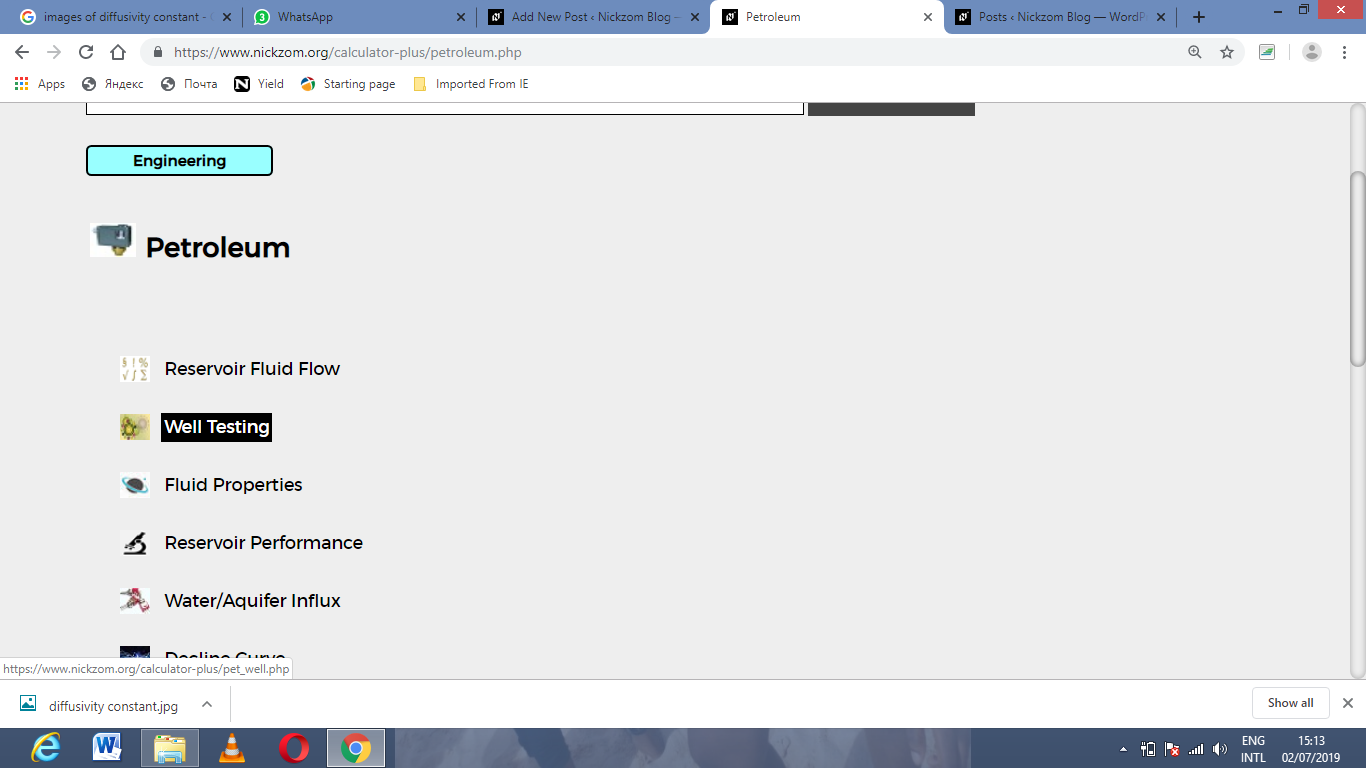Now, Click on Dimensionless Time under Well Testing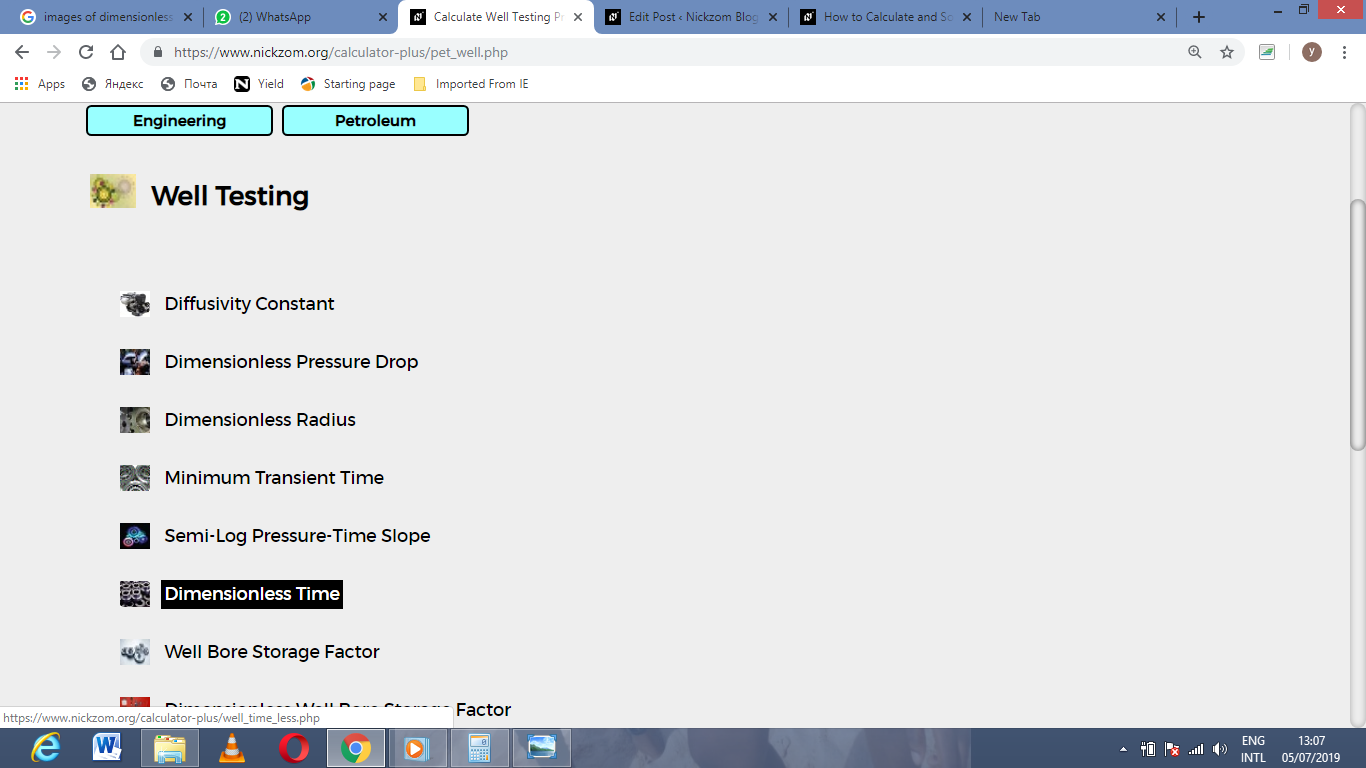The screenshot below displays the page or activity to enter your value, to get the answer for the dimensionless time according to the respective parameter which are the permeability (k), porosity (φ), viscosity (μ), well radius (rw), minimum transient time (t) and total compressibility (CT).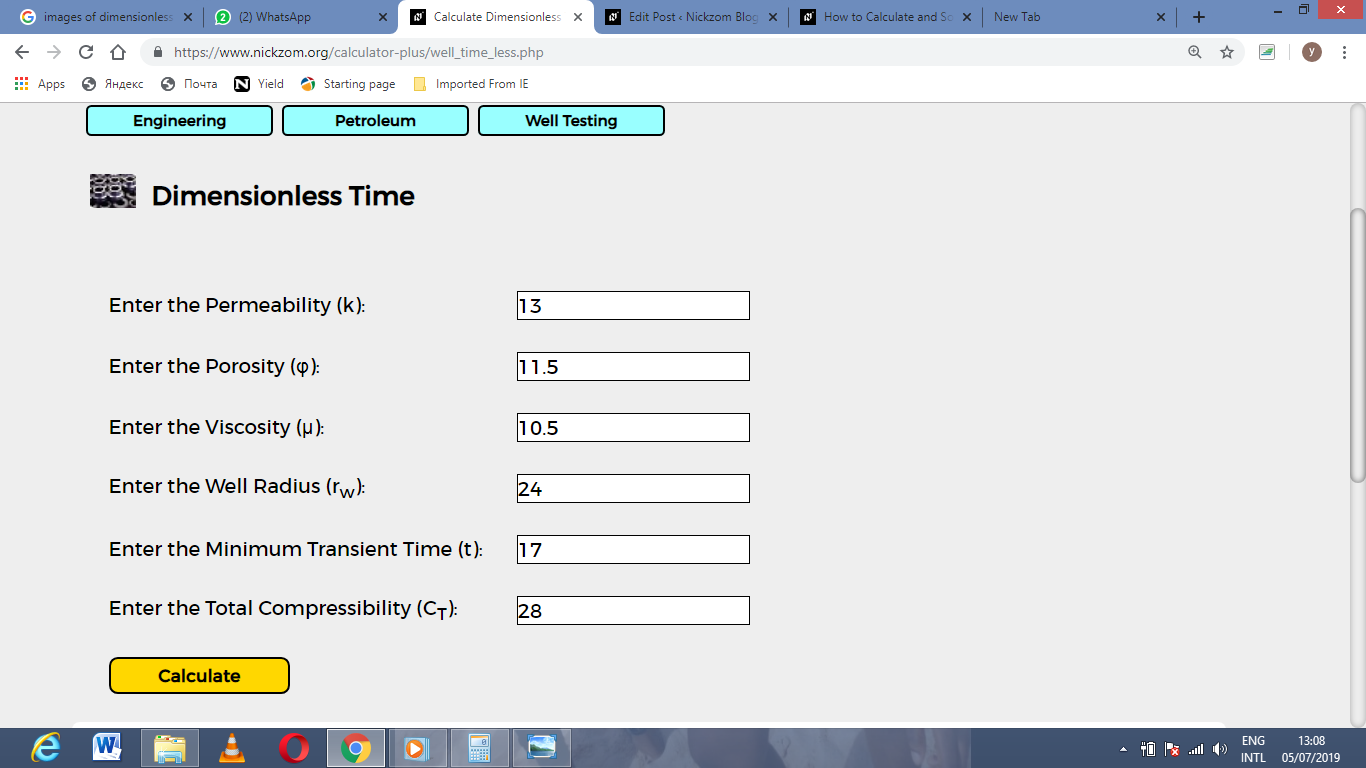Now, enter the value appropriately and accordingly for the parameter as required by the permeability (k) is 13, porosity (φ) is 11.5, viscosity (μ) is 10.5, well radius (rw) is 24, minimum transient time (t) is 17 and total compressibility (CT) is 28.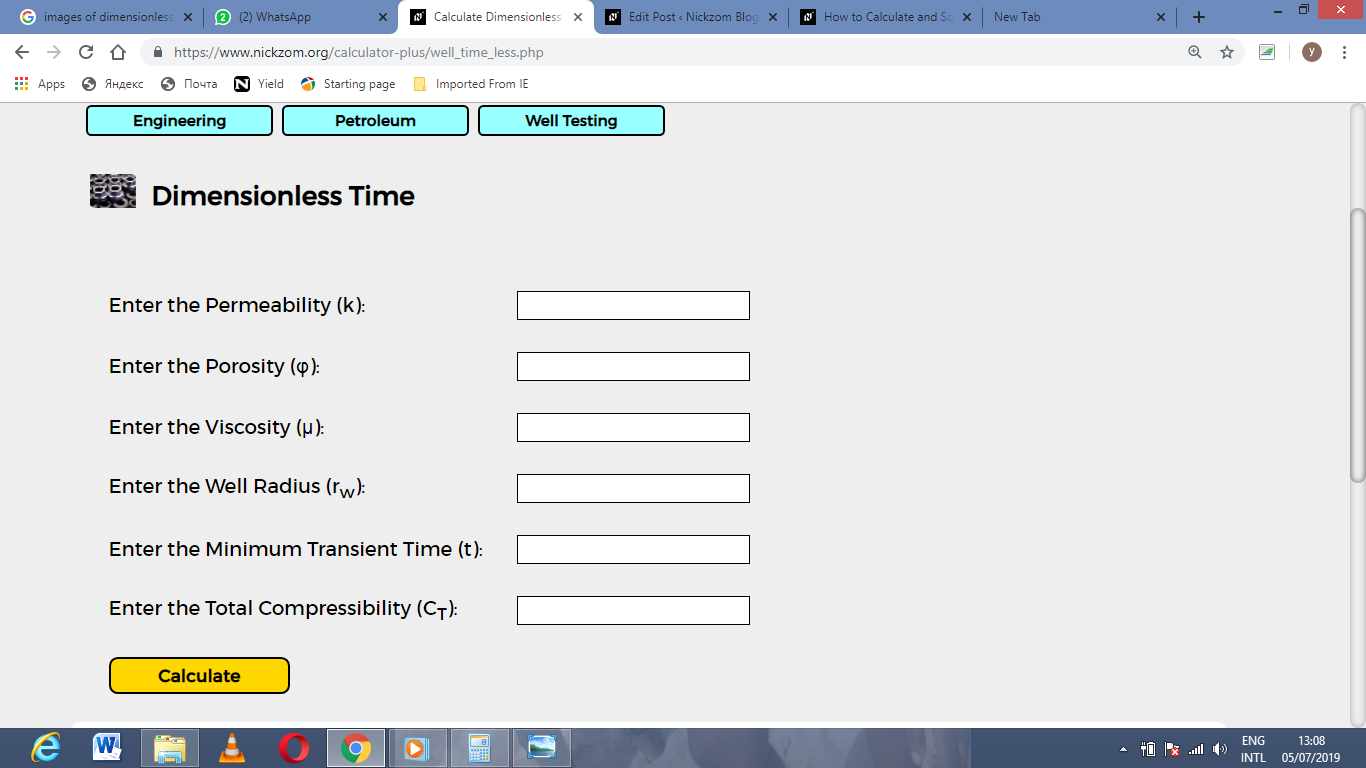Finally, Click on Calculate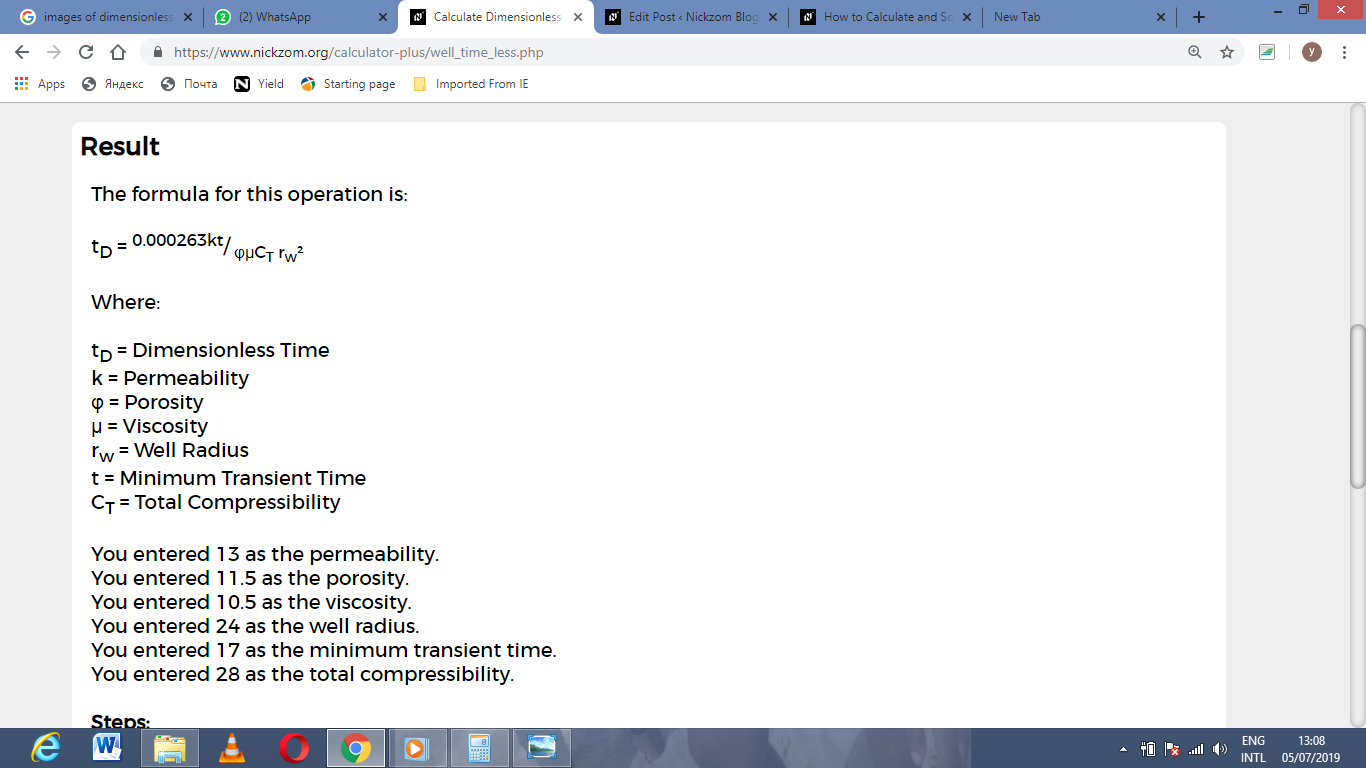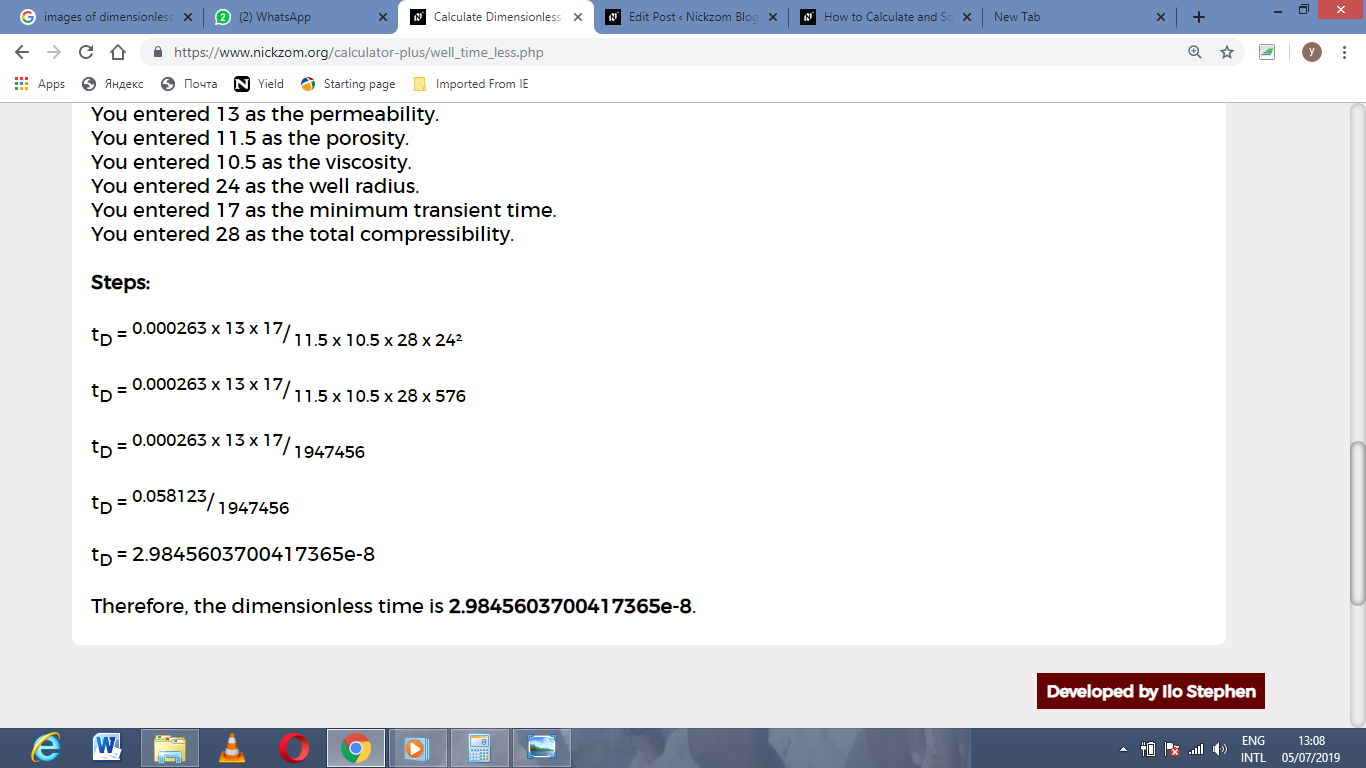As you can see from the screenshot above, Nickzom Calculator– The Calculator Encyclopedia solves for the dimensionless time and presents the formula, workings and steps too.

## How to Calculate and Solve for Minimum Transient Time in Well Testing | The Calculator Encyclopedia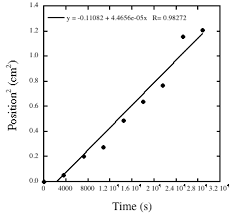The image above represents minimum transient time.

To compute for the minimum transient time, five essential parameters are needed and these parameters are permeability (k), porosity (φ), reservoir radius (r), viscosity (μ) and total compressibility (CT).

The formula for calculating the minimum transient time:

t = 9.48 x 104 φ CT r² / k

Where;

t = Minimum Transient Time
k = Permeability
φ = Porosity
μ = Viscosity
CT = Total Compressibility

Let’s solve an example;
Given that the permeability is 11, porosity is 14, reservoir radius is 7, viscosity is 13 and total compressibility is 22.
Find the minimum transient time?

This implies that;

k = Permeability = 11
φ = Porosity = 14
r = Reservoir Radius = 7
μ = Viscosity = 13
CT = Total Compressibility = 22

t = 9.48 x 104 φ CT r² / k
t = 9.48 x 104 x 14 x 22 x 7² / 11
t = 9.48 x 104 x 14 x 22 x 49 / 11
t = 1430721600 / 11
t = 130065600

Therefore, the minimum transient time is 13006500.

## How to Calculate and Solve for Diffusivity Constant | Well Testing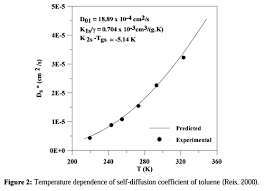The image above represents diffusivity constant.

To compute for the diffusivity constant, four essential parameters are needed and these parameters are pemerability (k), porosity (φ), viscosity (μ) and total compressibility (CT).

The formula for calculating diffusivity constant:

η = 0.000263k / φμCT

Where;

η = Diffusivity Constant
k = Permeability
φ = Porosity
μ = Viscosity
CT = Total Compressibility

Let’s solve an example;
Find the diffusivity constant where the permeability is 12, porosity is 9, viscosity is 7 and total compressibility is 11.

This implies that;

k = Permeability = 12
φ = Porosity = 9
μ = Viscosity = 7
CT = Total Compressibility = 11

η = 0.000263k /  φμCT
η = 0.000263 x 12 / 9 x 7 x 11
η = 0.003156 / 693
η = 0.000004554112554112554

Therefore, the diffusivity constant is 0.000004554112554112554.

Calculating the Permeability when the Diffusivity Constant, Porosity, Viscosity and Total Compressibility is Given.

k = n x φμCT / 0.000263

Where;

k = Permeability
η = Diffusivity Constant
φ = Porosity
μ = Viscosity
CT = Total Compressibility

Let’s solve an example;
Find the permeability where the diffusivity constant is 24, porosity is 10, viscosity is 5 and total compressibility is 13.

This implies that;

η = Diffusivity Constant = 24
φ = Porosity = 10
μ = Viscosity = 5
CT = Total Compressibility = 13

k = n x φμCT / 0.000263
k = 24 x 10 x 5 x 13 / 0.000263
k = 15600 / 0.000263
k = 59315589.3536

Therefore, the permeability is 59315589.3536.

Calculating the Porosity when the Diffusivity Constant, Permeability, Viscosity and Total Compressibility is Given.

φ = 0.000263k / nμCT

Where;

φ = Porosity
k = Permeability
η = Diffusivity Constant
μ = Viscosity
CT = Total Compressibility

Let’s solve an example;
Find the porosity where the diffusivity constant is 28, permeability is 8, viscosity is 12 and total compressibility is 18.

This implies that;

k = Permeability = 8
η = Diffusivity Constant = 28
μ = Viscosity = 12
CT = Total Compressibility = 18

φ = 0.000263k / nμCT
φ = 0.000263 x 8 / 28 x 12 x 18
φ = 0.002104 / 6048
φ = 3.478e-7

Therefore, the porosity is 3.47e-7.

## How to Calculate and Solve for Gas Initially in Place of a Reservoir Fluid Flow | The Calculator Encyclopedia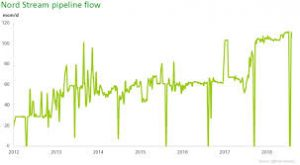The image above represents gas initially in place.

To compute for gas initially in place, five essential parameters are needed and these parameters are drainage area (A), initial gas FVF (Bgi), initial water saturation (Swi), porosity (φ) and thickness (h).

The formula for calculating gas initially in place:

G = 43560Ah φ (1 – Swi) / Bgi

Where;

G = Gas Initially in Place
A = Drainage Area
Bgi = Initial Gas FVF
Swi = Initial Water Saturation
φ = Porosity
h = Thickness

Let’s solve an example;
Find the gas initially in place with a drainage area of 44, initial gas FVF of 23, initial water saturation of 32, porosity of 18 and exponent of 14.

This implies that;

A = Drainage Area = 44
Bgi = Initial Gas FVF = 23
Swi = Initial Water Saturation = 32
φ = Porosity = 18
h = Thickness = 14

G = 43560Ah φ (1 – Swi) / Bgi
G = 43560 x 44 x 14 x 18 (1 – 32) / 23
G = 43560 x 44 x 14 x 18 (-31) / 23
G = -14972791680 / 23
G = -650990942.6

Therefore, the gas initially in place is -650990942.6

## Nickzom Calculator Solves Porosity in Sonic Log under Geology – The Calculator Encyclopedia

According to Wikipedia,

Sonic logging is a well logging tool that provides a formation’s interval transit time, designated as, which is a measure of a formation’s capacity to transmit seismic waves. Geologically, this capacity varies with lithology and rock textures, most notably decreasing with an increasing effective porosity

Nickzom Calculator+ (Professional Version) – The Calculator Encyclopedia solves calculations on porosity for sonic log and shows the steps carefully.

The parameters to input to get the answer for your porosity problem are:

• Internal Transit Time of Formation
• Internal Transit Time of Matrix
• Internal Transit Time of the Fluid in the Well Bore
• Compaction Factor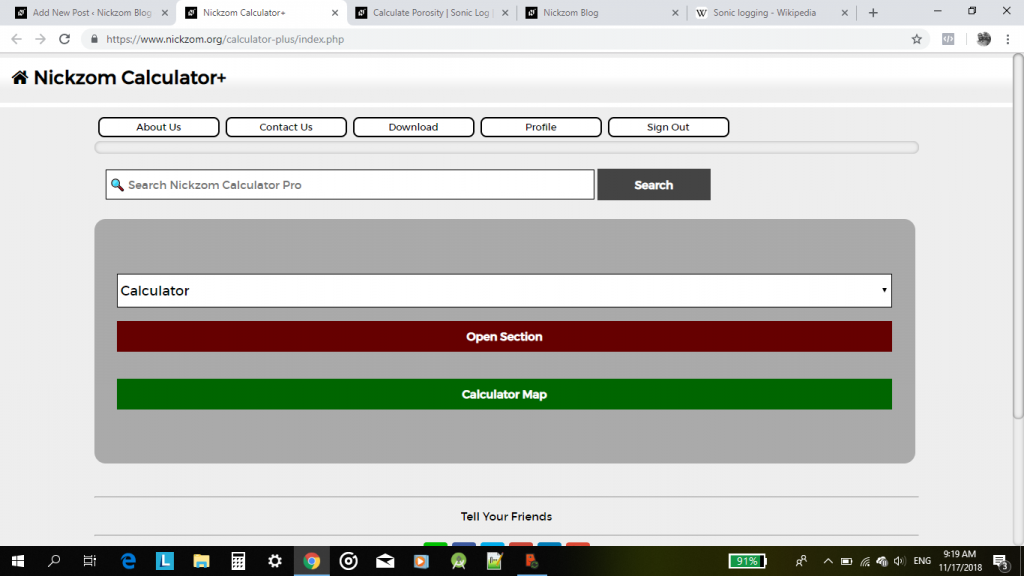Select Geology from the drop down.